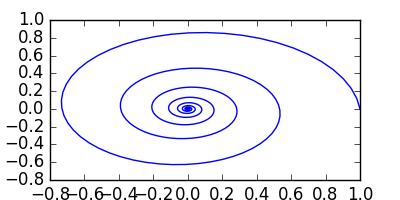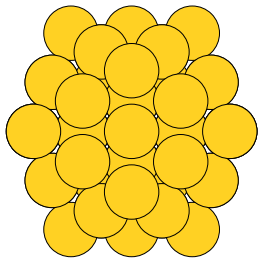## Sending html emails from org-mode with org-mime

| categories: | tags:

On the org-mode mailing list there was some discussion about sending html mail using orgmode. The support for this in mu4e is deprecated. There is the org-mime library though, and it supports a lot of what is needed for this. As I played around with it though, I came across some limitations:

1. I found equations were not rendered as images in the html, and files (in links) were not attached out of the box. I fixed that here.
2. I found it useful to modify the org-mime commands to leave point in the To: field when composing emails from org-buffers.
3. For use with mu4e, I created a function to open a message in org-mu4e-compose-org-mode, and added a C-cC-c hook to allow me to send it easily (here).

This post documents some work I did figuring out how to send html mails. After some testing, some of these should probably be patched in org-mime.

First, you need to require this library.

(require 'org-mime)


You can send a whole org buffer in html like with this command: org-mime-org-buffer-htmlize. Not all of the internal links work for me (at least in gmail).

The default behavior leaves you at the end of the buffer, which is not too nice. We lightly modify the function here to leave in the To: field.

(defun org-mime-org-buffer-htmlize ()
"Create an email buffer containing the current org-mode file
exported to html and encoded in both html and in org formats as
mime alternatives."
(interactive)
(org-mime-send-buffer 'html)
(message-goto-to))


## 1 From an org-headline in an org-file

You can compose an email as an org-heading in any org-buffer, and send it as html. In an org-heading, you need to specify a MAIL_FMT property of html, e.g.:

   :PROPERTIES:
:MAIL_FMT: html
:END:


Note the following properties can also be set to modify the composed email.

           (subject (or (funcall mp "MAIL_SUBJECT") (nth 4 (org-heading-components))))
(to (funcall mp "MAIL_TO"))
(cc (funcall mp "MAIL_CC"))
(bcc (funcall mp "MAIL_BCC"))


Then, send it with org-mime-subtree

Here I modify this function to leave me in the To: field.

(defun org-mime-subtree ()
"Create an email buffer containing the current org-mode subtree
exported to a org format or to the format specified by the
MAIL_FMT property of the subtree."
(interactive)
(org-mime-send-subtree
(or (org-entry-get nil "MAIL_FMT" org-mime-use-property-inheritance) 'org))
(message-goto-to))


Here are some sample elements to see if they convert to html reasonably.

### 1.1 Markup

bold

underlined

italics

strikethrough

code

Subscripts: H2O Superscripts: H+ An entity: To ∞ and beyond

### 1.2 Equations

$$x^2$$

$x^4$

$$e^x$$

### 1.3 Tables

 x y 1 2

### 1.4 Lists

A nested list.

• one
• Subentry under one.
• two

A definition list:

def1
first definition

A checklist:

• [ ] A checkbox

Here is a numbered list:

1. number 1
2. number 2

### 1.5 Code block

import numpy as np
import matplotlib.pyplot as plt

t = np.linspace(0, 10)
x = np.cos(t) * np.exp(-t)
y = np.sin(t) * np.exp(-t)

plt.plot(x, y)
plt.savefig('spiral.png')### 1.6 An image from somewhere other than this directory### 1.7 Citations with org-ref

 a b c

See Table table-1.

# Bibliography

## 2 In a mail message

You might prefer to do this directly in an email. Here is how you can do it in mu4e. I use this command to open a message in org-mode. The mode switches if you are in the header, or in the body. If you always do this, you could use a hook instead on message-mode. I do not want default html so I do not do it.

(defun mu4e-compose-org-mail ()
(interactive)
(mu4e-compose-new)
(org-mu4e-compose-org-mode))


For sending, we will use org-mime to htmlize it, and add a C-c C-c hook function to send it. This hook is a little tricky, we want to preserve C-c C-c behavior in org-mode, e.g. in code blocks, but send it if there is no other C-c C-c action that makes sense, so we add it to the end of the hook. Alternatively, you could bind a special key for it, or run the special command. Note the C-c C-c hook only works in the body of the email. From the header, a plain text message is sent.

(defun htmlize-and-send ()
"When in an org-mu4e-compose-org-mode message, htmlize and send it."
(interactive)
(org-mime-htmlize)
(message-send-and-exit)))



Here is a way to do this for non-mu4e users. It doesn't have the nice mode switching capability though, so you lose completion in emails, and header specific functions. You can switch back to message-mode to regain those.

(defun compose-html-org ()
(interactive)
(compose-mail)
(message-goto-body)
(setq *compose-html-org* t)
(org-mode))

(defun org-htmlize-and-send ()
"When in an org-mu4e-compose-org-mode message, htmlize and send it."
(interactive)

(when *compose-html-org*
(setq *compose-html-org* nil)
(message-mode)
(org-mime-htmlize)
(message-send-and-exit)))



## 3 Equations and file attachments do not seem to work out of the box

$$e^{i\pi} - 1 = 0$$

Out of the box, org-mime does not seem to attach file links to emails or make images for equations..

Here is an adaptation of org-mime-compose that does that for html messages.

(defun org-mime-compose (body fmt file &optional to subject headers)
(require 'message)
(let ((bhook
(lambda (body fmt)
(let ((hook (intern (concat "org-mime-pre-"
(symbol-name fmt)
"-hook"))))
(if (> (eval (length ,hook)) 0)
(with-temp-buffer
(insert body)
(goto-char (point-min))
(eval (run-hooks ',hook))
(buffer-string))
body))))
(fmt (if (symbolp fmt) fmt (intern fmt)))
(when (string= (org-element-property :type link) "file")
(message-goto-body)
(cond
((eq fmt 'org)
(require 'ox-org)
(insert (org-export-string-as
(org-babel-trim (funcall bhook body 'org)) 'org t)))
((eq fmt 'ascii)
(require 'ox-ascii)
(insert (org-export-string-as
(concat "#+Title:\n" (funcall bhook body 'ascii)) 'ascii t)))
((or (eq fmt 'html) (eq fmt 'html-ascii))
(require 'ox-ascii)
(require 'ox-org)
;; we probably don't want to export a huge style file
(org-export-htmlize-output-type 'inline-css)
(org-html-with-latex 'dvipng)
(html-and-images
(org-mime-replace-images
(org-export-string-as (funcall bhook body 'html) 'html t)))
(images (cdr html-and-images))
(html (org-mime-apply-html-hook (car html-and-images))))
(insert (org-mime-multipart
(org-export-string-as
(org-babel-trim
(funcall bhook body (if (eq fmt 'html) 'org 'ascii)))
(if (eq fmt 'html) 'org 'ascii) t)
html)
(mapconcat 'identity images "\n")))))
(mapc #'mml-attach-file files)))


## 4 Summary

This makes it pretty nice to send rich-formatted html text to people.